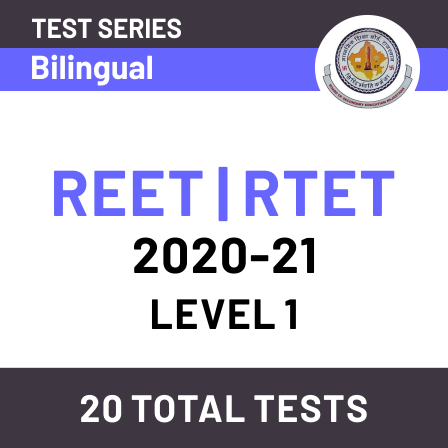Latest Teaching jobs   »   Shapes And Spatial Understanding- Download Mathematics...

# Shapes And Spatial Understanding- Download Mathematics Study Notes Free PDF For REET ExamMathematics is an equally important section for  REETMPTET & DSSSB Exams and has even more abundant importance in some other exams conducted by central or state govt. Generally, there are questions asked related to basic concepts and properties of the Shapes And Spatial Understanding.

To let you make the most of Mathematics section, we are providing important facts related to the Shapes And Spatial Understanding. At least 2-3 questions are asked from geometry topic in most of the teaching exams. We wish you all the best of luck to come over the fear of the Mathematics section.

How to Overcome Exam Fever, Especially When You Fear Maths

## SHAPES AND SPATIAL UNDERSTANDING

This unit can help young children build maths skills by encouraging them to explore and compare shapes and spatial relationships.

SPATIAL RELATIONSHIPS

Spatial Relationships explore the concept of where objects are in relationship to something else. Some spatial concepts are:

(a) Above, below
(b) Before, after
(c) High, low
(d) Small, big
(e) Outside, inside
(f) On top of, under
(g) Near, far
(h) In front of, in back of, behindIf we see above group (a), (b), (c) and (d) carefully, then we get the following results:
(a) a is bigger than b.
(b) a is inside and b is outside
(c) b is above and a is below.
(d) b is between a and c and a is far from c and is near to be.

Get free Study material for REET Exam

OPEN AND CLOSED CURVES

A curve with end points or the ends didn’t join up is called open curve.
A curve that joins up so there are no end point is called closed curve.Polygon: A closed plane figure made up of several line segments that are joined together. The sides do not cross each other. Exactly two sides meet at every vertex.
Some Polygons are-Polygon Parts:
Side- one of the line segments that’s make up the polygon.
Vertex- point where two sides meet. Two or more of these.
Diagonal- a line connecting two vertices that is not a side.
Interior angle- Angle formed by two adjacent sides inside the polygon.
Exterior Angle- Angled formed by two adjacent sides outside the polygon.

Mathematics Study Notes For All Teaching ExamsExample: Which one of the following figure is an open curve?Practice REET Subject wise Quiz

SOLID AROUND US
We live in a three-dimensional world. Every object you can see or touch has three dimensions that can be measured: length, width, and height.
Rectangular Room: The room you are sitting in can be described by these three dimensions such as L, B and H.
Dice: In a game of ludo, dice is in shape of cube.
Soccer ball: A soccer ball is a shape of sphere.
Conical Cape: A magician person or birthday cap are of conical shape.
Example: If Ram have three marbles of radius. If all marbles are same in colour and size, then total volume will be
(a) 4πr³ cubic unit
(b) 4/3 πr³ cubic unit
(c) 3πr² cubic unit
(d) ¾ πr³d cubic unit## Download Mathematics PDF Notes in Hindi

You May Also like to Read:Thank You, Your details have been submitted we will get back to you.